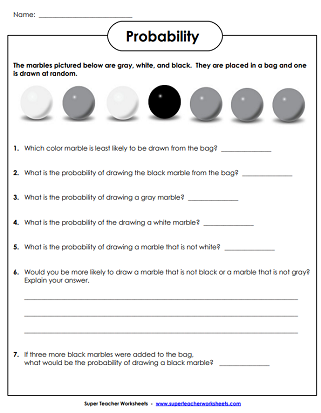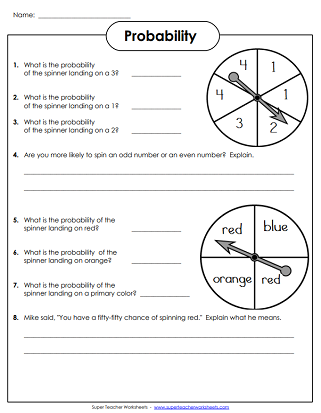# Probability Worksheets

These printable math worksheets will help students learn about probability of random events.Use the pictures of the spinners to determine the probability of outcomes for events.
Determine the probability of drawing certain marbles at random from a bag.
Color the marble pictures.  Then, write the probability of drawing certain colored marbles from a bag.
Slightly more advanced spinner pictures with probability questions.
Determine the probability of drawing particular letter tiles from a bag.
Answer the probability questions related to the prize wheel and the letter cube.
What is the probability of choosing a particular card from a deck?  Requires basic knowledge of standard playing cards.
Determine the probability of each scenario given.
Try this spinner experiment to test the mathematical and experimental probability of spinning diamonds, spades, clubs, or hearts on a spinner.
Try this coin-flipping experiment to test your hypothesis on probability.
Determine the mathematical probability and experimental probability of color outcomes on the spinner.
Nine spinners you can use for various probability activities and experiments.

## Also on Super Teacher Worksheets...

Basic Fraction Worksheets

Learn basic fraction skills with these printables

## Sample Worksheet ImagesMy Account
Site Information